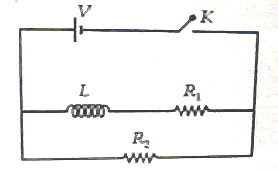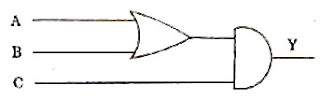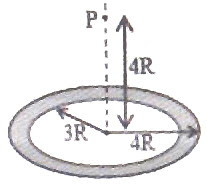## Tuesday, April 27, 2010

### All India Engineering/Architecture Entrance Examination 2010 (AIEEE 2010) Questions Involving Electromagnetic Induction

The following interesting multiple choice questions involving electromagnetic induction were included in the AIEEE 2010 question paper:(1) A rectangular loop has a sliding connector PQ of length l and resistance R Ω and it is moving with a speed v as shown. The set-up is placed in a uniform magnetic field going into the plane of the paper. The three currents I1, I2 and I are

(1) I1 = I2 = Blv/6R, I = Blv/3R

(2) I1 = I2 = Blv/R, I = 2Blv/R

(3) I1 = I2 = Blv/3R, I = 2Blv/3R

(4) I1 = I2 = I = Blv/R

The emf induced in the sliding connector PQ is ε = Blv, which is shown as a battery of internal resistance R in the adjoining figure.

The two external resistances R and R appear in parallel with this battery. The effective external resistance is therefore R/2 and the battery has to drive the current I through a total resistance R + (R/2), which is 3R/2Therefore, I = ε/(3R/2) = 2Blv/3R.

The current I gets divided equally between the two identical branches and hence I1 = I2 = Blv/3R.

(2) In the circuit shown below, the key K is closed at t = 0. The current through the battery is

(1) V(R1 + R2)/R1R2 at t = 0 and V/R2 at t = ∞

(2) VR1R2/√(R12+ R22) at t = 0 and V/R2 at t = ∞

(3) V/R2 at t = 0 and V(R1 + R2)/R1R2 at t =

(4) V/R2 at t = 0 and VR1R2/√(R12+ R22) at t =The current through the inductance branch is zero at the instant the circuit is switched on. [Remember the exponential growth of current in an LR circuit]

Therefore, at the instant t = 0 the current is limited by R2 alone and is equal to V/R2.

At t = ∞ the current has become steady, there is no induced voltage to oppose the flow of current and the inductor functions as a piece of conductor. The current is now limited by R1 and R2 which are in parallel with the battery. The current in this case is V/[R1R2/(R1 + R2)] = V(R1 + R2)/R1R2, as given in option (3).

You will find similar useful multiple choice question (with solution) here.

## Tuesday, April 20, 2010

### AIPMT 2010 Multiple Choice Questions (MCQ) on Electronics

Questions on electronics at the level expected from you in most of the entrance examinations are generally simple. (You won't find questions in this section in IIT-JEE and AP Physics Exam question papers). Here are the five questions on electronics (including digital circuits) which appeared in the AIPMT 2010 question paper:

(1) A common emitter amplifier has a voltage gain of 50, an input impedance of 100 Ω and an output impedance of 200 Ω. The power gain of the amplifier is

(1) 50

(2) 500

(3) 1000

(4) 1250

In the case of common emitter amplifier, the voltage gain Av is given by

Av = βac(Ro/Ri) where βac is the a.c. current gain, Ro is the output resistance and Ri is the intput resistance. (Instead of Ro and Ri you may use Zo and Zi, the respective impedances). The negative sign just indicates the 180º phase shift of the output signal with respect to the input signal.

Therefore we have 50 = βac×200/100 from which βac = 25.

Power gain = current gain×voltage gain = 25×50 = 1250.

(2) Which one of the following bonds produces a solid that reflects light in the visible region and whose electrical conductivity decreases with temperature and has high melting point?

(1) Covalent bonding

(2) Metallic bonding

(3) van der Waal's bonding

(4) Ionic bonding

This is a very simple question and the answer is metallic bonding.

(3) The device that can act as a complete electronic circuit is

(1) Zener diode

(2) Junction diode

(3) Integrated circuit

(4) Junction transistor

This too is a very simple question and the answer is integrated circuit.

(4) Which one of the following statement is FALSE ?

(1) The resistance of intrinsic semiconductor decreases with increase of temperature

(2) Pure Si doped with trivalent impurities gives a p-type semiconductor

(3) Majority carriers in a n-type semiconductor are holes

(4) Minority carries in a p-type semiconductor are electrons

The FALSE statement is given in option (3).

(5) To get an output Y = 1 from the circuit shown below, the input must be---A B C---

(1) 1 0 0

(2) 0 1 0

(3) 0 0 1

(4) 1 0 1

The correct option is (4) since the circuit implements the operation Y = (A+B).C

You will find the same question and some other questions on digital circuits with solution here: Karnataka CET Questions (MCQ) on Digital Circuits

## Wednesday, April 14, 2010

### IIT-JEE 2010 Questions on Gravitation

I would never die for my belief because I might be wrong.

– Bertrand Russell

Two questions on gravitation were included in the IIT-JEE 2010 question paper. They are given below with solution. The first question is a ‘single correct choice type’ (single answer type multiple choice) question where as the second question is an ‘integer type’ question which has a single digit integer ranging from 0 to 9 as answer.(1) A thin uniform annular disc (see figure) of mass M has outer radius 4R and inner radius 3R. The work required to take a unit mass from point P on its axis to infinity is

(A) (2GM /7R) (42 5)

(B) (2GM /7R) (42 5)

(C) GM /4R

(D) (2GM /5R) (2 – 1)

The work required to take a unit mass from infinity to point P is the gravitational potential at P and it will be negative. Therefore, the work required to take a unit mass from point P to infinity will be numerically equal to the gravitational potential at P but it will be positive.

The annular disc can be considered to be made of a large number of concentric rings of radii ranging from 3R to 4R. Consider one such ring of radius r and small thickness dr. Its mass dm is given by

dm = [M/π(16R2 – 9R2)] 2πr dr = 2Mrdr/7R2

If the rim of the ring is at distance x from the point P, the gravitational potential (dV) at P due to the ring is given by

dV = Gdm/x = – (2GM/7R2)(rdr/x)

But x = (16R2 + r2)1/2 and so we have dx= (½ )×(16R2 + r2)1/2 ×2rdr

Or, dx = rdr/(16R2 + r2)1/2 = rdr/x

Therefore dV = – (2GM/7R2)dx

The gravitational potential at P due to the entire annular disc is given by

V = dV = (2GM/7R2)dx = – (2GM/7R2)[x]

The integration is between the appropriate limits of x, which are (16R2 + 9R2)1/2 and (16R2 + 16R2)1/2, on substituting the values r = 3R and r = 4R respectively in the expression, x = (16R2 + r2)1/2. Therefore we have

V = – (2GM/7R2)[(16R2 + 16R2)1/2 (16R2 + 9R2)1/2]

= – (2GM/7R2)[R√(32) R√(25)]

Thus V = (2GM/7R) (42 5)

The negative sign is to be dispensed with to obtain the work required to take a unit mass from point P to infinity and the correct choice is (2GM/7R) (42 5).

(2) Gravitational acceleration on the surface of a planet is (√6/11)g, where g is the gravitational acceleration on the surface of the earth. The average mass density of the planet is 2/3 times that of the earth. If the escape speed on the surface of the earth is taken to be 11 kms–1, the escape speed on the surface of the planet in kms–1 will be :

[This is is an ‘integer type’ question. You have to work out this question and the single digit integer answer is to be shown in the Objective Response Sheet (ORS) by darkening the appropriate bubble].

We have vesc = √(2gR) where g is the acceleration due to gravity and R is the radius of the planet (or earth or star).

Therefore vesc α √(gR).

If gp and ge are the accelerations due to gravity on the surface of the planet and the earth, Rp and Re are their radii, and gp and ge the escape velocities respectively, we have

vp/ve = √( gpRp)/ √( geRe) = √[( gp/ge)(Rp/Re)] ………(i)

Since the gravitational acceleration on the surface is given by g = GM/R2 where G is the gravitational constant and M is the mass of the planet (or earth or star), we can write

g = G[(4/3)πR3ρ] /R2 where ρ is the average mass density of the planet (or earth or star).

Therefore g α and gp/ge = (Rp/Re)(ρp/ρe).

From this Rp/Re = (gp/ge)/(ρp/ρe).

But gp/ge = √6/11 and ρp/ρe = 2/3 as given in the question. Therefore we have

Rp/Re = (√6/11)×3/2

Substituting these in Eq(i),

vp/ve = √[(√6/11) (√6/11)×3/2] = (√6/11) ×√(3/2) = 3/11

Since ve = 11 kms–1, the escape speed on the surface of the planet in kms–1 will be

vp = 11×(3/11) = 3 kms–1

## Wednesday, April 07, 2010

### AIPMT 2010 Questions on Friction

The following multiple choice questions involving friction were included in the AIPMT 2010 question paper:(1) A block of mass m is in contact with the cart C as shown in the figure. The coefficient of static friction between the block and the cart is μ. The acceleration α of the cart that will prevent the block from falling statisfies

(1) α < g/μ

(2) α > mg/μ

(3) α > g/μm

(4) α g/μ

When the cart moves forwards with acceleration α, the block of mass m presses against the vertical surface of the cart with a horizontal force of magnitude mα. Therefore, the normal force acting on the block is mα and the maximum frictional force trying to prevent the block from falling is μmα. This must be greater than or equal to the weight mg of the block.

Therefore we have

μmα ≥ mg from which α g/μ

(2) A gramophone record is revolving with an angular velocity ω. A coin is placed at a distance r from the centre of the record. The static coefficient of friction is μ. The coin will revolve with the record if

(1) r μg/ω2

(2) r = μ2

(3) r < ω2/μg

(4) r μg/ω2

Let the mass of the coin be m. The coin will revolve with the record if the frictional force which supplies the centripetal force (required for the circular motion of the coin) is sufficient. Since the maximum value of the frictional force is μmg, we have

μmg mrω2

Therefore r μg/ω2.

You will find more questions (with solution) on friction on this site. One of the posts on friction is

Similar useful posts in this section can be seen